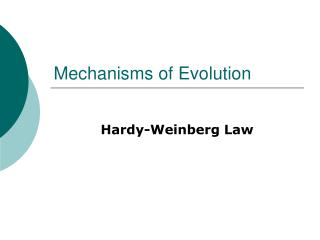Download PresentationMechanisms of Evolution

Mechanisms of Evolution - PowerPoint PPT Presentation

Mechanisms of Evolution. Hardy-Weinberg Law. Hardy-Weinberg Law. The Hardy–Weinberg principle states that the genotype frequencies in a population remain constant or are in equilibrium from generation to generation unless specific disturbing influences are introduced. Hardy-Weinberg Law.I am the owner, or an agent authorized to act on behalf of the owner, of the copyrighted work described.
Download PresentationMechanisms of Evolution

Download Policy: Content on the Website is provided to you AS IS for your information and personal use and may not be sold / licensed / shared on other websites without getting consent from its author.While downloading, if for some reason you are not able to download a presentation, the publisher may have deleted the file from their server.

- - - - - - - - - - - - - - - - - - - - - - - - - - E N D - - - - - - - - - - - - - - - - - - - - - - - - - -
Presentation Transcript
1. Mechanisms of Evolution Hardy-Weinberg Law

2. Hardy-Weinberg Law • TheHardy–Weinberg principle • states that the genotype frequencies in a population remain constant or are in equilibrium from generation to generation unless specific disturbing influences are introduced.

3. Hardy-Weinberg Law • Hardy–Weinberg equilibrium is an ideal state that provides a baseline against which change can be analyzed

4. Hardy-Weinberg Law • Genotype -This is the "internally coded, inheritable information" carried by all living organisms. • "blueprint" or set of instructions for building and maintaining a living creature.

5. Hardy-Weinberg Law • Population – all members of a species living in one locale • The occurrence of evolution within a population is established by determining that the genetic makeup of the population has changed.

6. Hardy-Weinberg Law Most common conditions that cause a change in gene pool frequencies • Mutation • Gene flow • Nonrandom mating • Size of population • Natural selection

7. Hardy-Weinberg Law • Mutations Changes in the way cells function or develop, caused by an inherited genetic defect or environmental exposure.

8. Hardy-Weinberg Law • Gene flowthe natural transfer of genes from one population into the genetic makeup of another population through hybridization and interbreeding • migration or the dispersal of seeds and pollen

9. Hardy-Weinberg Law • Non-random mating-Mating that has not occurred due to chance, and therefore has had human interference

10. Hardy-Weinberg Law Population Size Population must of a sufficient size so that the laws of probability apply

11. Hardy-Weinberg Law • Natural selection – occurs when a new variation arises that allows certain members of a population to capture more resources.

12. Hardy-Weinberg Law • Equation for calculating gene pool frequencies • p2 + 2pq + q2 = 1

13. Hardy-Weinberg Law • p2= frequency of AA • ( homozygous dominant) • Two dominant alleles • 2pq= frequency of Aa • (heterozygous) • Two different alleles one dominant, one recessive • q2= frequency of aa • ( homozygous recessive) two recessive alleles

14. Hardy-Weinberg Law • Remember: • Dominant phenotype characteristics are displayed by both: • Homozygous dominant & heterozygous genotypes • ( homozygous dominant) • Two dominant alleles • (heterozygous) • Two different alleles one dominant, one recessive

15. Hardy-Weinberg Law • Example: Determine the Population that is heterozygous for white coat • *ALLELE FOR BLACK COAT IS RECESSIVE

16. Hardy-Weinberg Law • 12 white • 4 black 1. CALCULATE q2 *Count the individuals that are homozygous recessive *calculate the percentage of the total population they represent. THIS IS q2 4 of the 16 cats show the recessive phenotype which is 25% or 0.25 q2 =.25

17. Hardy-Weinberg Law • 12 white • 4 black 2. Find q Take the square root of q2 to obtain q, the frequency of the recessive allele q=.5

18. Hardy-Weinberg Law • 12 white • 4 black 3. Find p The sum of the frequencies of both alleles =100% We know q=.5 So if p+q=1 ( a population in genetic equilibrium) Then p = .5

19. Hardy-Weinberg Law • 12 white • 4 black 4. Find 2pq The frequency of the heterozygotes is 2pq which is the percent of the population that is heterozygous for white coat: 2pq= 2(.5) (.5) = .50 50% is heterozygous for white coat

20. Hardy-Weinberg Law • In class today/homework: • Finding a frequency of certain allele. • Specific allele/ total population of alleles

21. Hardy-Weinberg Law • WHY is this important???????? • Based on these equations, we can determine useful but difficult-to-measure facts about a population. http://www.mnn.com/earth-matters/animals/blogs/100-years-later-the-passenger-pigeon-still-haunts-us#ixzz3Bc4dEeCH

22. Hardy-Weinberg Law • WHY is this important???????? • Cancer • Autism • Downs syndrome